# D/A conversion

PESIT, BSCEvery time I think of LD!

## FUn ! :-) :-)

[ yes, that's possible! ]

Happy times ahead . . .## D/a converter

Wanna see something cool?

Go down!

A sneak-peak with Dr. Collin

Let's get started!

## weight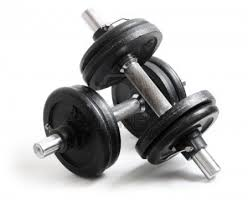??

## 3-bit binary signal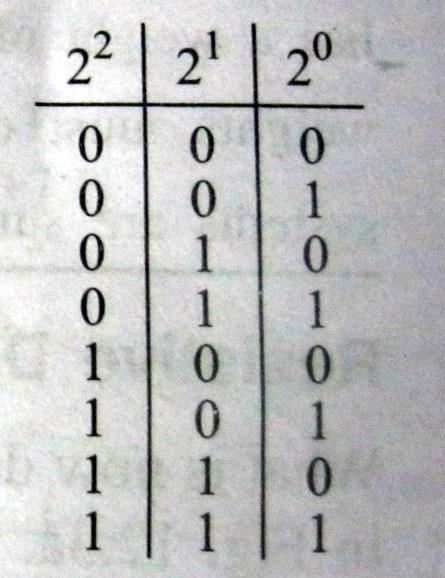Points to remember!

• Sum of binary weights should be equal to one. (1)• General formula for binary weights associated with LSB:Time for a question?

Q. What's the binary equivalent   weight                                                of  each bit in a 4-bit system?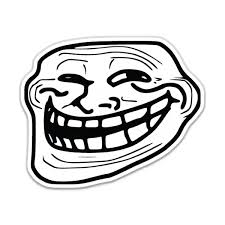Hint: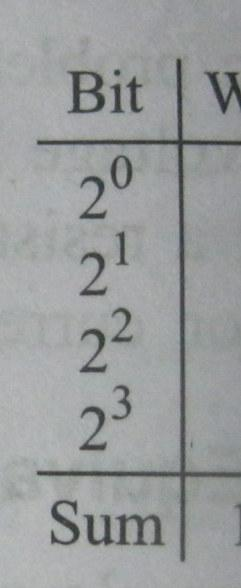Answer: 1/15 , 2/15, 4/15 and 8/15

## Resistive DividersWhat should it produce? Exactly this: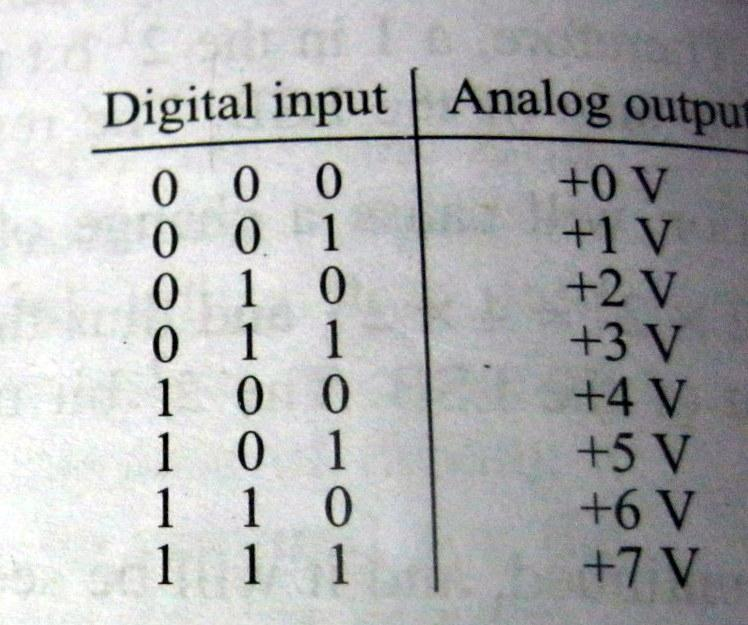## How does it work?

Nothing unusual, the resistors come into play!1. Convert LSB(s) to their analog value. ( 001 = 1, 010 =2)
2. Sum up these analog values.
simple enough, right? ;-)

## Milliman's theorem

``"The voltage between the ends of the circuit is equal to the total current entering the supernode divided by the total equivalent conductance of the supernode". ``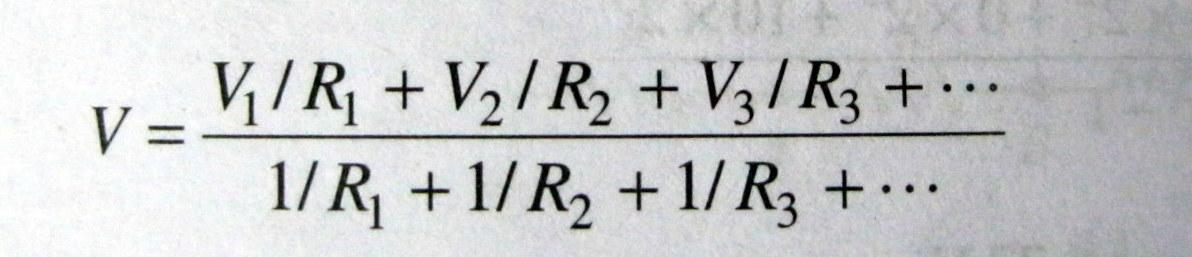Apply the formula for the circuit above.

???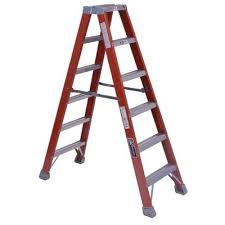Resistive network!

## Watch This!

A 3-bit DAC in action. :-)

### Lets continue with ladder networks...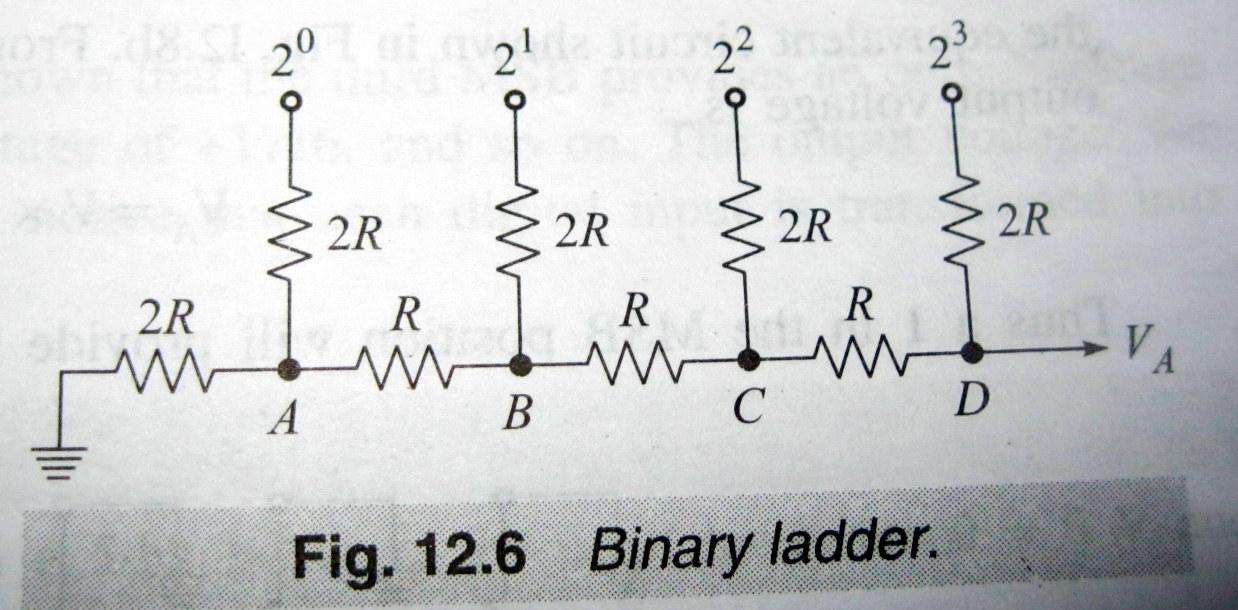### Now, we bring in the

OP-AMPsAny idea why?

Obvious, isn't it!

to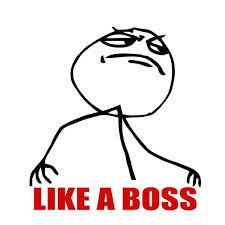## by now,## Relax with recap!

1. Binary weights

2. Resistive dividers

AND, WE'RE DONE!

But before that,

What's the basic difference between analog and digital signals?

Relax! You don't have to answer this one.

## Resources

1. Find the slides here.
2. Search for more here.
3. Great help from our text by Donald P Leach and co.

A presentation made by Abhiram Ravikumar.
(just in case you forgot! ;-) )